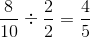# SSAT Middle Level Math : How to add fractions

## Example Questions

### Example Question #444 : Concepts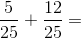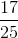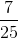Explanation:

Add the numerators and leave the denominator the same: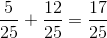Answer:### Example Question #445 : Concepts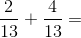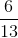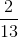Explanation:

Add the numerators and leave the denominator the same: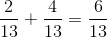Answer:### Example Question #52 : How To Add Fractions

The time is now 10:38 PM. What time will it be in two hours and fifty-two minutes?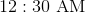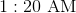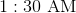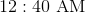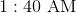Explanation:

It is now 10:38 PM. In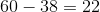minutes, it will be 11:00 PM; in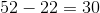minutes, the minutes will read 30, so the time will be 11:30 PM. In another two hours, the hours will read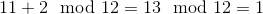,

so the time will be 1:30 AM.

### Example Question #53 : How To Add Fractions

Solve the expression.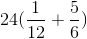None of theseExplanation:

Start by solving for the term in parenthesis. We will need to find the least common denominator in order to add the fractions. Since 6 can be multiplied by 2 to get 12, 12 must be the least common denominator.

Convert the fractions so that they share a denominator of 12.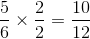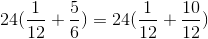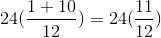Multiply.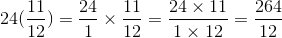You can then simplify the fraction be removing 12 from both the numerator and denominator.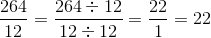### Example Question #54 : How To Add Fractions

Which of the following is the sum of five-sevenths and one-half?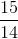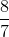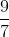The correct answer is not among the other responses.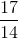Explanation:

Since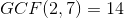, express each fraction as its equivalent in fourteenths, and add the numerators, as follows: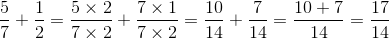### Example Question #55 : How To Add Fractions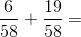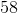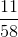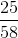Explanation:

Add the numerators and keep the denominator the same: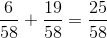Answer:### Example Question #61 : How To Add Fractions

Which of the following is the sum of seven tenths and seventeen hundredths?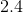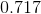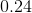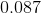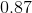Explanation:

Seven tenths is equal to 0.7; seventeen hundredths is equal to 0.17. Add them, rewriting 0.7 as 0.70: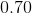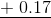### Example Question #62 : How To Add Fractions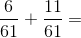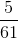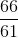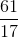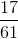Explanation:

Add the numerators and leave the denominators the same: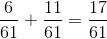### Example Question #63 : How To Add Fractions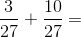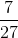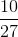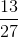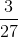Explanation:

To solve, add the numerators and leave the denominators the same: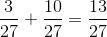### Example Question #731 : Numbers And Operations

Joe paited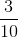of the fence an Sara painted. How much of the fence is painted?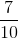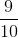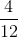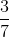Explanation: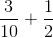In order to solve this problem, we first need to make common denominators.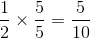Now that we have common denominators, we can add the fractions. Remember, when we add fractions, the denominator stays the same, we only add the numerator.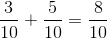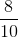can be reduced be dividing both sides by.